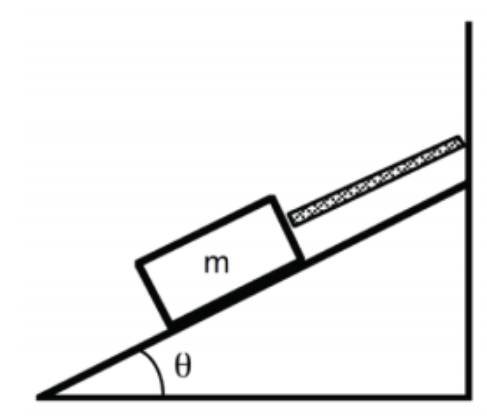# Problem: A 20 N crate is held at rest on an incline by a rope that is parallel to the incline as shown on the figure to the right. The incline is 30° above the horizontal. The coefficient of static friction, μs, between the crate and the incline is 0.35. Write down two separate equations for Newton's second law with static friction: one for forces pointing along the ramp and one for forces pointing perpendicular to the ramp. Do some forces appear in only one of Newton's law equations? For forces that have some component in both, make sure you break down those force vectors correctly in the equation.

###### FREE Expert Solution

In this problem, we are required to set up newton's second law along the x and y axes.

Newton's second law:

84% (343 ratings)###### Problem Details

A 20 N crate is held at rest on an incline by a rope that is parallel to the incline as shown on the figure to the right. The incline is 30° above the horizontal. The coefficient of static friction, μs, between the crate and the incline is 0.35.Write down two separate equations for Newton's second law with static friction: one for forces pointing along the ramp and one for forces pointing perpendicular to the ramp. Do some forces appear in only one of Newton's law equations? For forces that have some component in both, make sure you break down those force vectors correctly in the equation.